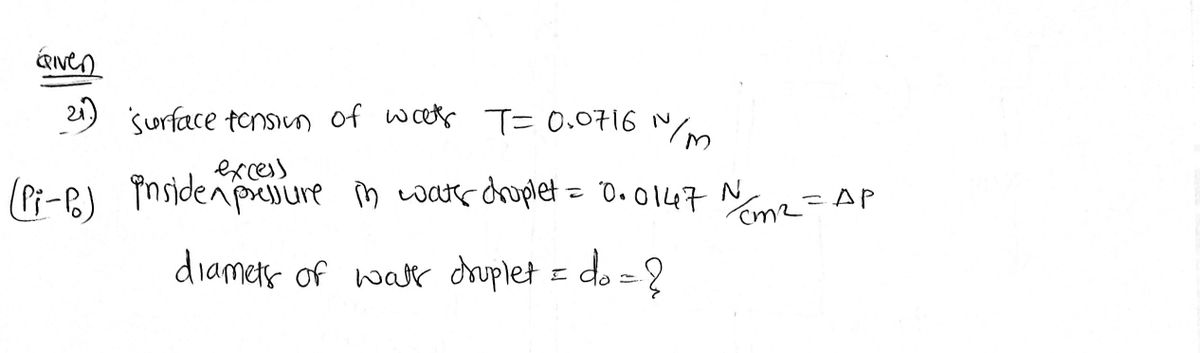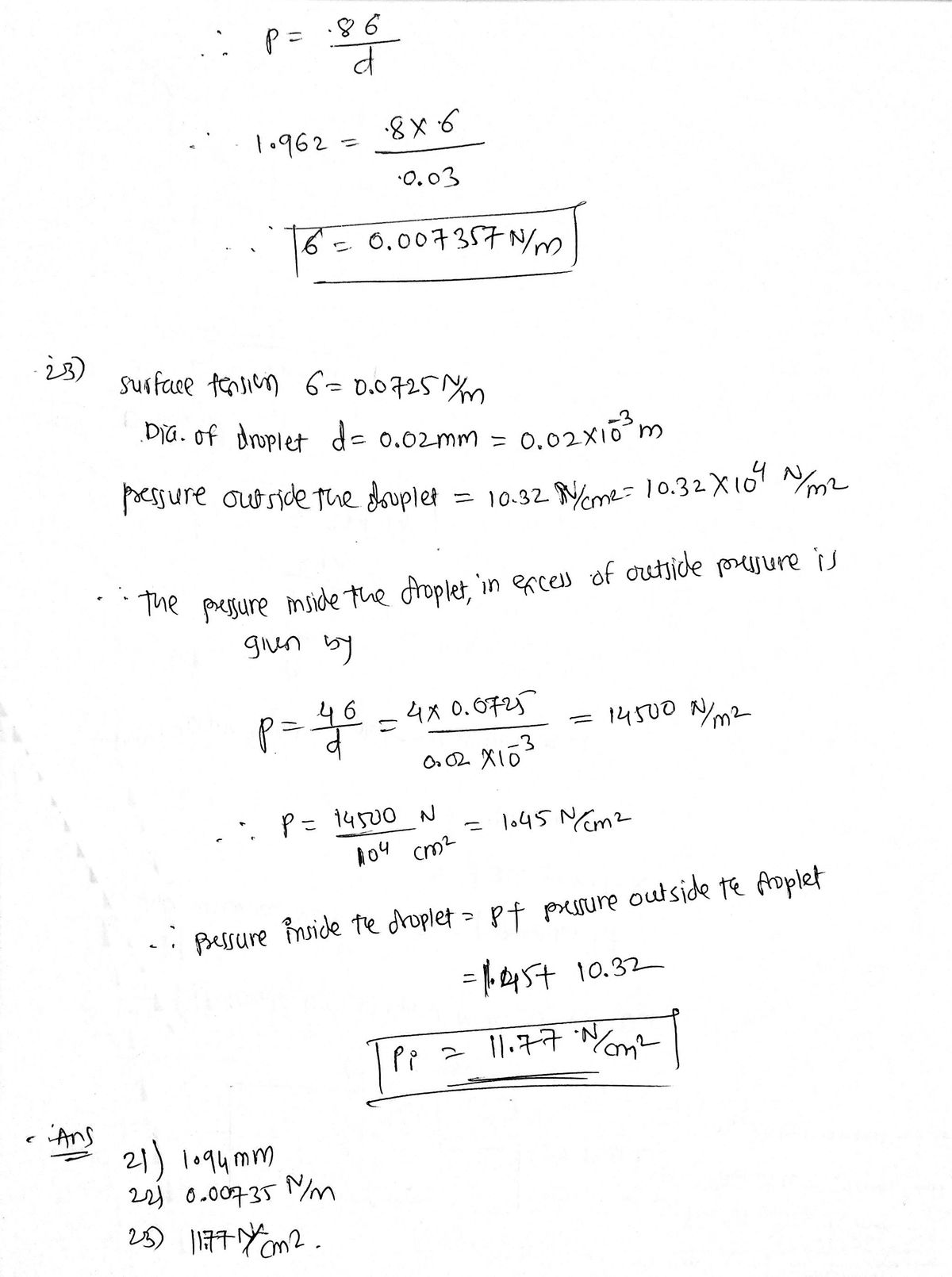# The surface tension of water in contact with air at 20°C is given as 0.0716 N/m. The pressure inside pressure droplet of water is to be 0.0147 N/cm2 greater than the outside pressure, calculate the diameter of the droplet of water. Find the surface tension in a soap bubble of 30 mm diameter when the inside pressure is 1.962 N/m² above atmosphere. The surface tension of water in contact with air is given as 0.0725 N/m. The pressure outside the droplet of water of diameter 0.02 mm is atmospheric (10.32 N/cm2). Calculate the pressure within the droplet of water. -cm2-greater-than-the-outside-pressure-calculate-t

Question-AnswerCategory: Fluid MechanicsThe surface tension of water in contact with air at 20°C is given as 0.0716 N/m. The pressure inside pressure droplet of water is to be 0.0147 N/cm2 greater than the outside pressure, calculate the diameter of the droplet of water. Find the surface tension in a soap bubble of 30 mm diameter when the inside pressure is 1.962 N/m² above atmosphere. The surface tension of water in contact with air is given as 0.0725 N/m. The pressure outside the droplet of water of diameter 0.02 mm is atmospheric (10.32 N/cm2). Calculate the pressure within the droplet of water. -cm2-greater-than-the-outside-pressure-calculate-t

The surface tension of water in contact with air at 20°C is given as 0.0716 N/m. The pressure inside pressure droplet of water is to be 0.0147 N/cm2 greater than the outside pressure, calculate the diameter of the droplet of water. Find the surface tension in a soap bubble of 30 mm diameter when the inside pressure is 1.962 N/m² above atmosphere. The surface tension of water in contact with air is given as 0.0725 N/m. The pressure outside the droplet of water of diameter 0.02 mm is atmospheric (10.32 N/cm2). Calculate the pressure within the droplet of water.